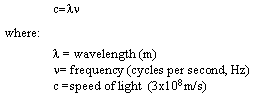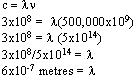## Search

##### In This Section

As was noted in the previous section, the first requirement for remote sensing is to have an energy source to illuminate the target (unless the sensed energy is being emitted by the target). This energy is in the form of electromagnetic radiation.[Text Version]

All electromagnetic radiation has fundamental properties and behaves in predictable ways according to the basics of wave theory. Electromagnetic radiation consists of an electrical field(E) which varies in magnitude in a direction perpendicular to the direction in which the radiation is traveling, and a magnetic field (M) oriented at right angles to the electrical field. Both these fields travel at the speed of light (c).[Text Version]

Two characteristics of electromagnetic radiation are particularly important for understanding remote sensing. These are the wavelength and frequency.[Text Version]

The wavelength is the length of one wave cycle, which can be measured as the distance between successive wave crests. Wavelength is usually represented by the Greek letter lambda (λ). Wavelength is measured in metres (m) or some factor of metres such as nanometres (nm, 10-9 metres), micrometres (μm, 10-6 metres) (μm, 10-6 metres) or centimetres (cm, 10-2 metres). Frequency refers to the number of cycles of a wave passing a fixed point per unit of time. Frequency is normally measured in hertz (Hz), equivalent to one cycle per second, and various multiples of hertz.

Wavelength and frequency are related by the following formula:[Text Version]

Therefore, the two are inversely related to each other. The shorter the wavelength, the higher the frequency. The longer the wavelength, the lower the frequency. Understanding the characteristics of electromagnetic radiation in terms of their wavelength and frequency is crucial to understanding the information to be extracted from remote sensing data. Next we will be examining the way in which we categorize electromagnetic radiation for just that purpose.

## Did you know?

"I've Gone Batty!"...that remote sensing, in its broadest definition, includes ultrasounds, satellite weather maps, speed radar, graduation photos, and sonar - both for ships and for bats!. Hospitals use imaging technology, including CAT scans, magnetic resonance imaging (3-D imaging of soft tissue), and x-rays for examining our bodies. These are all examples of non-intrusive remote sensing methods.

...you can use an oscilloscope, a special electronic device which displays waves similar to the electromagnetic radiation waves you have seen here, to look at the wavelength and frequency patterns of your voice. High-pitched sounds have short wavelengths and high frequencies. Low sounds are the opposite. Scientists say that the Earth itself vibrates at a very low frequency, making a sound far below the human hearing range.

...that the concept of wavelength and frequency is an important principle behind something called the Doppler Shift, which explains how sound and light waves are perceived to be compressed or expanded if the object producing them is moving relative to the sensor. As a train or race car advances towards us, our ears tend to hear progressively lower sounds or frequencies (shorter wavelengths) until it reaches us, the original frequency of the object when it is broadside, then even lower frequencies as it moves further away. This same principle (applied to light) is used by astronomers to see how quickly stars are moving away from us (the Red shift).

## Whiz quiz

The first requirement for remote sensing is an energy source which can illuminate a target. What is the obvious source of electromagnetic energy that you can think of? What "remote sensing device" do you personally use to detect this energy? The answer is ...Assume the speed of light to be 3x108 m/s. If the frequency of an electromagnetic wave is 500,000 GHz (GHz = gigahertz = 109 m/s), what is the wavelength of that radiation? Express your answer in micrometres(μm). The answer is ...

Answer 1: The most obvious source of electromagnetic energy and radiation is the sun. The sun provides the initial energy source for much of the remote sensing of the Earth surface. The remote sensing device that we humans use to detect radiation from the sun is our eyes. Yes, they can be considered remote sensors - and very good ones - as they detect the visible light from the sun, which allows us to see. There are other types of light which are invisible to us...but more about that later.[Text Version]

Answer 2: Using the equation for the relationship between wavelength and frequency, let's calculate the wavelength of radiation of a frequency of 500,000 GHz.